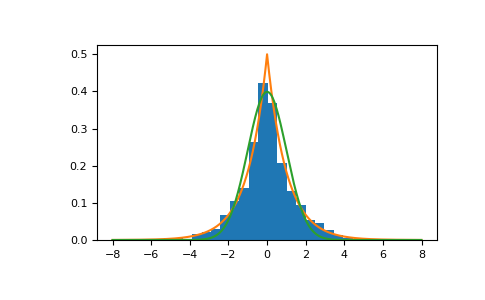# numpy.random.laplace¶

numpy.random.laplace(loc=0.0, scale=1.0, size=None)

Draw samples from the Laplace or double exponential distribution with specified location (or mean) and scale (decay).

The Laplace distribution is similar to the Gaussian/normal distribution, but is sharper at the peak and has fatter tails. It represents the difference between two independent, identically distributed exponential random variables.

Parameters: loc : float or array_like of floats, optional The position,, of the distribution peak. Default is 0. scale : float or array_like of floats, optional, the exponential decay. Default is 1. size : int or tuple of ints, optional Output shape. If the given shape is, e.g., (m, n, k), then m * n * k samples are drawn. If size is None (default), a single value is returned if loc and scale are both scalars. Otherwise, np.broadcast(loc, scale).size samples are drawn. out : ndarray or scalar Drawn samples from the parameterized Laplace distribution.

Notes

It has the probability density functionThe first law of Laplace, from 1774, states that the frequency of an error can be expressed as an exponential function of the absolute magnitude of the error, which leads to the Laplace distribution. For many problems in economics and health sciences, this distribution seems to model the data better than the standard Gaussian distribution.

References

 [R457460] Abramowitz, M. and Stegun, I. A. (Eds.). “Handbook of Mathematical Functions with Formulas, Graphs, and Mathematical Tables, 9th printing,” New York: Dover, 1972.
 [R458460] Kotz, Samuel, et. al. “The Laplace Distribution and Generalizations, ” Birkhauser, 2001.
 [R459460] Weisstein, Eric W. “Laplace Distribution.” From MathWorld–A Wolfram Web Resource. http://mathworld.wolfram.com/LaplaceDistribution.html
 [R460460] Wikipedia, “Laplace distribution”, http://en.wikipedia.org/wiki/Laplace_distribution

Examples

Draw samples from the distribution

>>> loc, scale = 0., 1.
>>> s = np.random.laplace(loc, scale, 1000)


Display the histogram of the samples, along with the probability density function:

>>> import matplotlib.pyplot as plt
>>> count, bins, ignored = plt.hist(s, 30, normed=True)
>>> x = np.arange(-8., 8., .01)
>>> pdf = np.exp(-abs(x-loc)/scale)/(2.*scale)
>>> plt.plot(x, pdf)


Plot Gaussian for comparison:

>>> g = (1/(scale * np.sqrt(2 * np.pi)) *
...      np.exp(-(x - loc)**2 / (2 * scale**2)))
>>> plt.plot(x,g)#### Previous topic

numpy.random.hypergeometric

#### Next topic

numpy.random.logistic